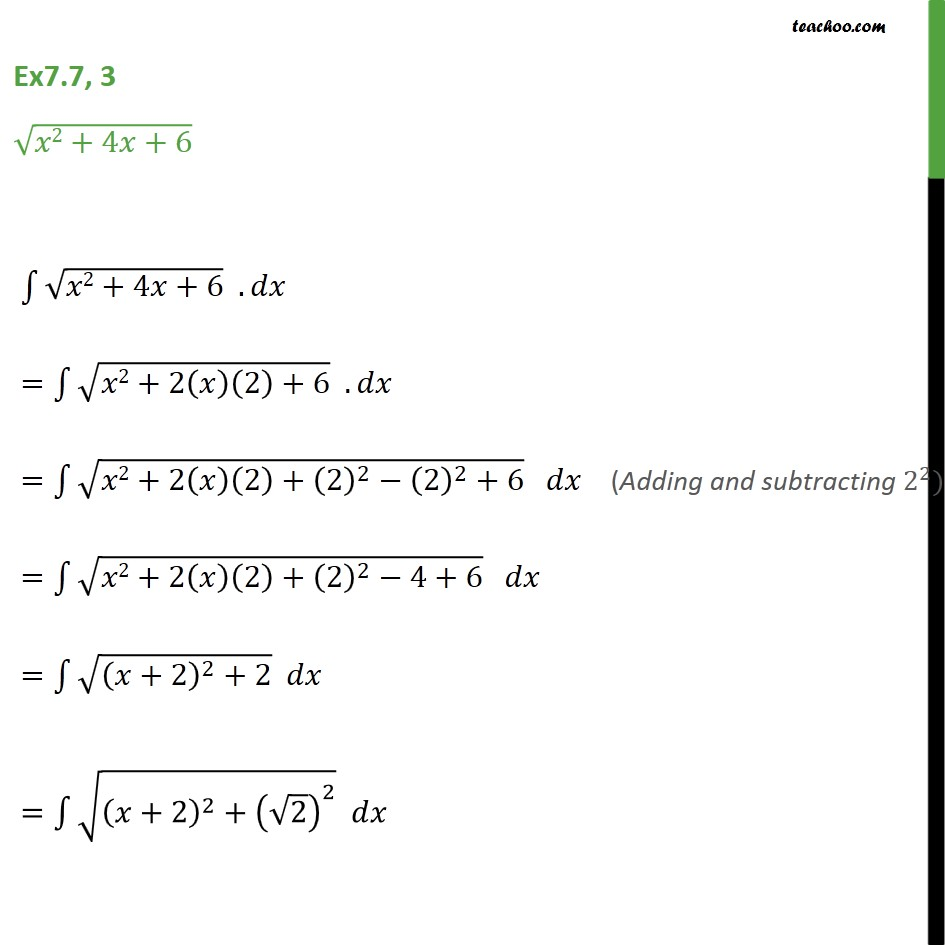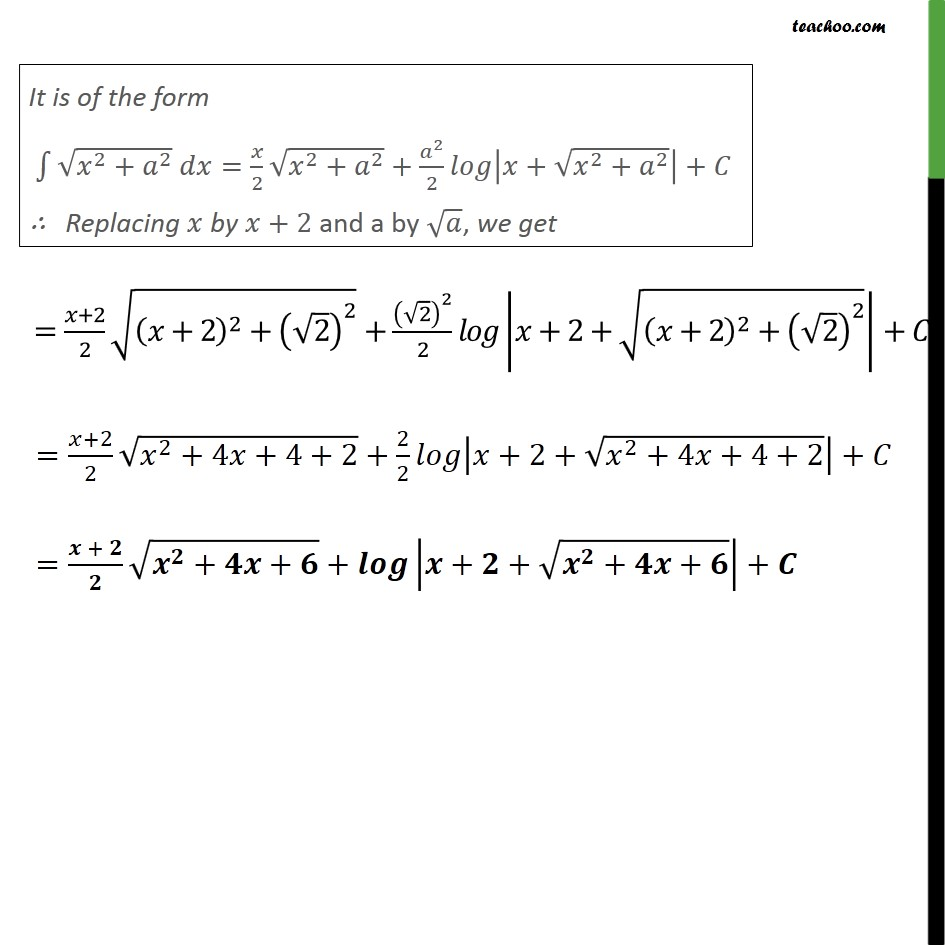Ex 7.7

Chapter 7 Class 12 Integrals
Serial order wiseLearn in your speed, with individual attention - Teachoo Maths 1-on-1 Class

### Transcript

Ex7.7, 3 2+4 +6 2+4 +6 . = 2+2 2 +6 . = 2+2 2 + 2 2 2 2 +6 = 2+2 2 + 2 2 4+6 = +2 2 +2 = +2 2 + 2 2 = +2 2 +2 2 + 2 2 + 2 2 2 +2+ +2 2 + 2 2 + = +2 2 2 +4 +4+2 + 2 2 +2+ 2 +4 +4+2 + = + + + + + + + + +